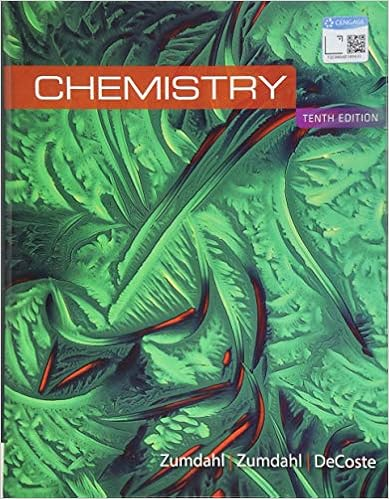# Ima chemist found the density of freon 11 cfcl 3 to

• Test Prep
• 19
• 88% (8) 7 out of 8 people found this document helpful

This preview shows page 8 - 10 out of 19 pages.

##### We have textbook solutions for you!
The document you are viewing contains questions related to this textbook.The document you are viewing contains questions related to this textbook.
Chapter 1 / Exercise 110
Chemistry
ZumdahlExpert Verified
44. Ima Chemist found the density of Freon-11 (CFCl3) to be 5.58 g/L under her experimental conditions. Her measurements showed that the density of an unknown gas was 4.38 g/L under the same conditions. What is the molar mass of the unknown? A) 96.7 g/mol B) 108 g/mol C) 127 g/mol D) 165 g/mol E) 175 g/mol Ans:
B Difficulty: M 45. A flask with a volume of 3.16 L contains 9.33 grams of an unknown gas at 32.0°C and 1.00 atm. What is the molar mass of the gas?
C Difficulty: M 46. Dr. I. M. A. Brightguy adds 0.1727 g of an unknown gas to a 125-mL flask. If Dr. B finds the pressure to be 736 torr at 20.0°C, is the gas likely to be methane, CH4, nitrogen, N2, oxygen, O2, neon, Ne, or argon, Ar?
E) O2
E Difficulty: M 47. A 250.0-mL sample of ammonia, NH3(g), exerts a pressure of 833 torr at 42.4°C. What mass of ammonia is in the container?
59.8 g
B Difficulty: M 48. What is the pressure in a 7.50-L flask if 0.15 mol of carbon dioxide is added to 0.33 mol of oxygen? The temperature of the mixture is 48.0°C. A) 0.252 atm B) 0.592 atm C) 1.69 atm D) 3.96 atm E) Ans:
4.80 atm
C Difficulty: M 49. If 0.750 L of argon at 1.50 atm and 177°C and 0.235 L of sulfur dioxide at 95.0 kPa and 63.0°C are added to a 1.00-L flask and the flask's temperature is adjusted to 25.0°C, what is the resulting pressure in the flask?
1.86 atm
C Difficulty: H
##### We have textbook solutions for you!
The document you are viewing contains questions related to this textbook.The document you are viewing contains questions related to this textbook.
Chapter 1 / Exercise 110
Chemistry
ZumdahlExpert Verified
Chapter 5: Gases and the Kinetic-Molecular Theory Page 74 50. A gas mixture consists of equal masses of methane (molecular weight 16.0) and argon (atomic weight 40.0). If the partial pressure of argon is 200. torr, what is the pressure of methane, in torr?
556 torr
D Difficulty: M 51. A gas mixture, with a total pressure of 300. torr, consists of equal masses of Ne (atomic weight 20.) and Ar (atomic weight 40.). What is the partial pressure of Ar, in torr?
B Difficulty: M 52. An unknown liquid is vaporized in a 273-mL flask by immersion in a water bath at 99°C. The barometric pressure is 753 torr. If the mass of the vapor retained in the flask is 1.362 g, what is its molar mass? A) 20.4 g/mol B) 40.9 g/mol C) 112 g/mol D) 154 g/mol E) 184 g/mol Ans:
D Difficulty: H 53. A gas consists of 85.7 % carbon and 14.3 % hydrogen, by weight. A sample of this gas weighing 0.673 g occupies 729 mL at a pressure of 720.0 mmHg and a temperature of 77°C. Calculate its empirical and molecular formulas.
2
4
6
6
4
B Difficulty: M
•••